Next: Efficiency Considerations Up: No Title Previous: Just One More

# Some Common Techniques

To become a skilled Prolog programmer requires, above all else, practice. However, there are a few standard ways of constructing a program which give some overall structure to the task. Some of these are described below:

tn:br   Recursion, decomposing a structure. The key idea is to separate base cases from recursive cases, then make sure that the recursive subgoals all tend towards one or more of the base cases. The general form of this technique is:

```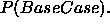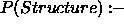```

For example:

```member(X, [X|_]).
member(X, [_|T]):-
member(X, T).
```

tn:rs   Recursion, building up a structure in recursive subgoals. Typically two arguments are used: the first storing the accumulated result and the second being used to "pass back" the final state of the accumulator.

```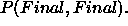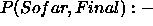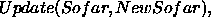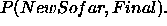```

For example:

```reverse([], Final, Final).
reverse([H|T], Sofar, Final):-
reverse(T, [H|Sofar], Final).
```

tn:rb   Recursion, building up a structure in the head of each clause. This normally involves just one extra argument which contains one or more variables to be instantiated from successful recursive subgoals.

``````

For example:

```numbers_in_term(A, [A]):- number(A).
numbers_in_term(A + B, Final):-
numbers_in_term(A, PA),
numbers_in_term(A, PB),
append(PA, PB, Final).
```

tn:cc   Committment by cases. If the problem is one which involves a number of mutually exclusive cases then these can be tackled using a sequence of clauses with cuts placed at the points of committment.

```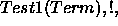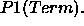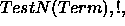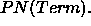```

For example:

```has_type(X, variable):-
var(X), !.
has_type(X, atom):-
atom(X), !.
has_type(X, number):-
number(X), !.
has_type(X, structure):-
nonvar(X),
functor(X, _, N), N > 0.
```

tn:fdl   Failure driven loops to force all solutions. This is often used in combination with printing routines.

```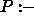Goal,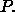```

For example:

```all_solutions(Goal):-
Goal,
write(Goal), nl,
fail.
all_solutions(_).
```

tn:rl   Repeat loops to perform some task until a terminating condition is obtained. These are often used as replacements for recursive predicates in cases where a simple cycle of repeating and testing is required.

```repeat,

Goal,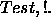```

For example:

```read_until(Prompt, Test):-
repeat,
write(Prompt), read(Answer),
((Test, !) ; fail).
```

tn:cf   Cut and fail to ensure no solutions on particular cases.

```UndesirableCase,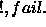OTHER DEFINITIONS OF P.```

For example:

```no_numbers([H|_]):-
number(H),
!, fail.
no_numbers([_|T]):-
no_numbers(T).
no_numbers([]).
```

tn:dn   Double negation to apply a test without binding any variables in the terms used during the test.

```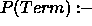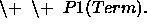```

For example:

```unifiable(Term1, Term2):-
\+ \+ Term1 = Term2.
```Next: Efficiency Considerations Up: No Title Previous: Just One More

Dave Stuart Robertson
Tue Jul 7 10:44:26 BST 1998